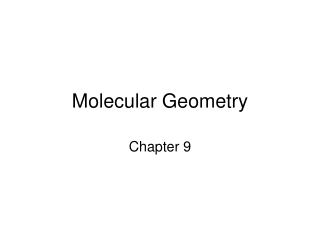# Molecular Geometry - PowerPoint PPT PresentationDownload PresentationMolecular Geometry

Molecular GeometryDownload Presentation## Molecular Geometry

- - - - - - - - - - - - - - - - - - - - - - - - - - - E N D - - - - - - - - - - - - - - - - - - - - - - - - - - -
##### Presentation Transcript

1. Molecular Geometry Chapter 9

2. Molecular Shapes The shape of a molecule plays an important role in its reactivity. By noting the number of bonding and nonbonding electron pairs we can easily predict the shape of the molecule.

3. What Determines the Shape of a Molecule? Simply put, electron pairs, whether they be bonding or nonbonding, repel each other. By assuming the electron pairs are placed as far as possible from each other because of Coulombic repulsion between electrons, we can predict the shape of the molecule.

4. Electron Domains We can refer to the electron pairs as electrondomains. In a double or triple bond, all electrons shared between those two atoms are on the same side of the central atom; therefore, they count as one electron domain. • This molecule has four electron domains.

5. Electron domain practice • How many total electron domains are there for the central atom? • How many are bonding? • How many are nonbonding?

6. Valence Shell Electron Pair Repulsion Theory (VSEPR) “The best arrangement of a given number of electron domains is the one that minimizes the repulsions among them.”

7. Electron-Domain Geometries These are the electron-domain geometries for two through six electron domains around a central atom.

8. Electron-Domain Geometries All one must do is count the number of electron domains in the Lewis structure. The geometry will be that which corresponds to that number of electron domains.

9. Electron domain geometry practice • What is the electron domain geometry for each of the following molecules?

10. Molecular Geometries The electron-domain geometry is often not the shape of the molecule, however. The molecular geometry is that defined by the positions of only the atoms in the molecules, not the nonbonding pairs.

11. Molecular Geometries Within each electron domain, then, there might be more than one molecular geometry.

12. Linear Electron Domain In this domain, there is only one molecular geometry: linear. NOTE: If there are only two atoms in the molecule, the molecule will be linear no matter what the electron domain is.

13. Trigonal Planar Electron Domain There are two molecular geometries: Trigonal planar, if all the electron domains are bonding and are equidistant from each other Bent, if one of the domains is a nonbonding pair. The nonbonding electrons repel each other and take up more space than the bonding electrons causing the shape to bend

14. Nonbonding Pairs and Bond Angle Nonbonding pairs are physically larger than bonding pairs. Therefore, their repulsions are greater; this tends to decrease bond angles in a molecule.

15. Multiple Bonds and Bond Angles Double and triple bonds place greater electron density on one side of the central atom than do single bonds. Therefore, they also affect bond angles.

16. Tetrahedral Electron Domain There are three molecular geometries: Tetrahedral, if all are bonding pairs Trigonal pyramidal if one is a nonbonding pair Triangle of atoms (3) + 1 atom for peak of pyramid Bent if there are two nonbonding pairs

17. Trigonal Bipyramidal Electron Domain There are four distinct molecular geometries in this domain: Trigonal bipyramidal Seesaw T-shaped Linear

18. Octahedral Electron Domain All positions are equivalent in the octahedral domain. There are three molecular geometries: Octahedral Square pyramidal Square planar

19. Larger Molecules In larger molecules, it makes more sense to talk about the geometry about a particular atom rather than the geometry of the molecule as a whole.

20. Larger Molecules This approach makes sense, especially because larger molecules tend to react at a particular site in the molecule. The shape of the molecule impacts its function (example: enzymes)

21. Electron domain geometry practice • What is the molecular geometry (VSEPR) structure for the following molecules?